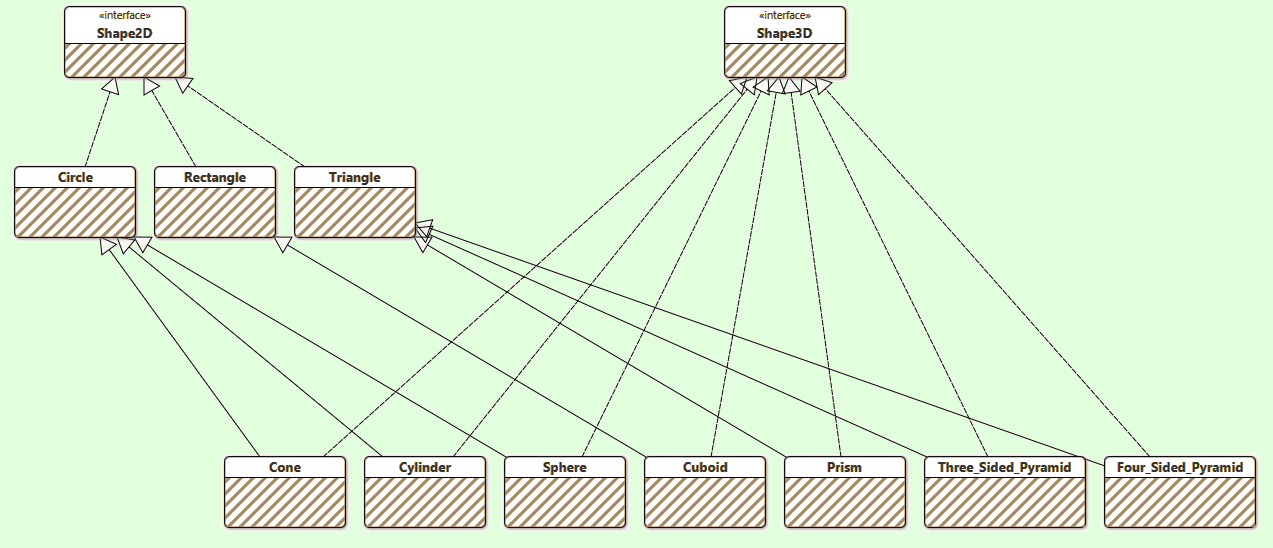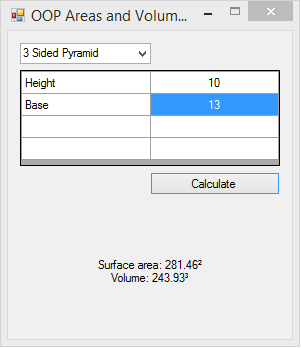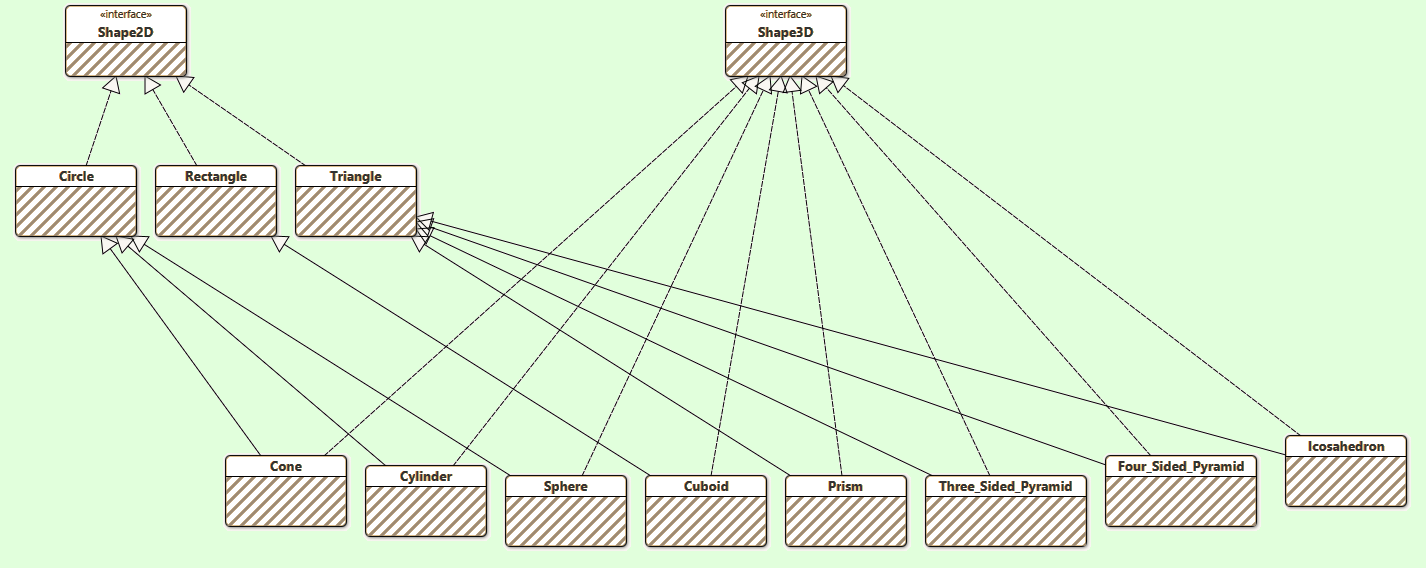#OOP Areas and VolumesThe existing Model diagram.

This is an OOP Areas and Volumes Calculator. It consists of a core containing ten classes representing different geometric shapes. These shapes fall into two categories - 2D and 3D. All of the 2D Shape Classes implement the Shape2D Interface. All of the 3D Classes extend one of the 2D Classes, therefore, they indirectly implement the Shape2D Interface. Additionally, all of the 3D Classes implement the Shape3D Interface.

• Circle
• Rectangle
• Triangle

## These are the 3D Classes

• Cone
• Cylinder
• Sphere
• Cuboid
• Prism
• Three_Sided_Pyramid
• Four_Sided_Pyramid

Implementing an Interface in a Class means that your Class must use the methods and functions stipulated in the Interface. It also means that any of the ten Core Shape Classes can be created as an Object of type Shape2D, and the seven 3D Core Shape Classes can be created as an Object of type Shape3D.

## The Shape2D Interface

```Public Interface Shape2D

Function getArea() As Decimal

End Interface
```

## The Shape3D Interface

```Public Interface Shape3D

Function getVolume() As Decimal

End Interface
```

## The Concrete Model Classes

### Circle

```''' <summary>
''' 2D Circle class
''' </summary>
Public Class Circle
Implements Shape2D

Public Const sortIndex As Integer = 0

End Sub

''' <summary>
''' Implemented getArea function
''' </summary>
''' <returns>Surface area of shape with specified dimensions</returns>
Public Overridable Function getArea() As Decimal Implements Shape2D.getArea
Return CDec(Math.PI * Me.Radius ^ 2)
End Function

End Class
```

### Rectangle

```''' <summary>
''' 2D Rectangle class
''' </summary>
Public Class Rectangle
Implements Shape2D

Public Const sortIndex As Integer = 1

Public Property Length As Decimal
Public Property Width As Decimal

Public Sub New(Length As Decimal, Width As Decimal)
Me.Length = Length
Me.Width = Width
End Sub

''' <summary>
''' Implemented getArea function
''' </summary>
''' <returns>Surface area of shape with specified dimensions</returns>
Public Overridable Function getArea() As Decimal Implements Shape2D.getArea
Return Me.Length * Me.Width
End Function

End Class
```

### Triangle

```''' <summary>
''' 2D Triangle class
''' </summary>
Public Class Triangle
Implements Shape2D

Public Const sortIndex As Integer = 2

Public Property SideA As Decimal
Public Property SideB As Decimal
Public Property SideC As Decimal

Public Sub New(SideA As Decimal, SideB As Decimal, SideC As Decimal)
Me.SideA = SideA
Me.SideB = SideB
Me.SideC = SideC
End Sub

''' <summary>
''' Implemented getArea function
''' </summary>
''' <returns>Surface area of shape with specified dimensions</returns>
Public Overridable Function getArea() As Decimal Implements Shape2D.getArea
Try
Dim s As Decimal = CDec((Me.SideA / 2) + (Me.SideB / 2) + (Me.SideC / 2))
Return CDec(Math.Sqrt(s * ((s - Me.SideA) * (s - Me.SideB) * (s - Me.SideC))))
Catch ex As exception
Return 0D
End Try
End Function

End Class
```

### Cone

```''' <summary>
''' 3D Cone class
''' </summary>
Public Class Cone
Inherits Circle
Implements Shape3D

Public Shadows Const sortIndex As Integer = 3

Public Property Height As Decimal

Public Sub New(Height As Decimal, Radius As Decimal)
Me.Height = Height
End Sub

''' <summary>
''' Overriden getArea function
''' </summary>
''' <returns>Surface area of shape with specified dimensions</returns>
Public Overrides Function getArea() As Decimal
End Function

''' <summary>
''' Implemented getVolume function
''' </summary>
''' <returns>Volume of shape with specified dimensions</returns>
Public Function getVolume() As Decimal Implements Shape3D.getVolume
Return CDec((Math.PI * MyBase.Radius ^ 2 * Me.Height) / 3)
End Function

End Class
```

### Cylinder

```''' <summary>
''' 3D Cylinder class
''' </summary>
Public Class Cylinder
Inherits Circle
Implements Shape3D

Public Shadows Const sortIndex As Integer = 4

Public Property Height As Decimal

Public Sub New(Height As Decimal, Radius As Decimal)
Me.Height = Height
End Sub

''' <summary>
''' Overriden getArea function
''' </summary>
''' <returns>Surface area of shape with specified dimensions</returns>
Public Overrides Function getArea() As Decimal
Return CDec(2 * Math.PI * MyBase.Radius * Me.Height + 2 * Math.PI * MyBase.Radius ^ 2)
End Function

''' <summary>
''' Implemented getVolume function
''' </summary>
''' <returns>Volume of shape with specified dimensions</returns>
Public Function getVolume() As Decimal Implements Shape3D.getVolume
Return CDec(Math.PI * MyBase.Radius ^ 2 * Me.Height)
End Function

End Class
```

### Sphere

```''' <summary>
''' 3D Sphere class
''' </summary>
Public Class Sphere
Inherits Circle
Implements Shape3D

Public Shadows Const sortIndex As Integer = 5

End Sub

''' <summary>
''' Overriden getArea function
''' </summary>
''' <returns>Surface area of shape with specified dimensions</returns>
Public Overrides Function getArea() As Decimal
Return CDec(4 * Math.PI * MyBase.Radius ^ 2)
End Function

''' <summary>
''' Implemented getVolume function
''' </summary>
''' <returns>Volume of shape with specified dimensions</returns>
Public Function getVolume() As Decimal Implements Shape3D.getVolume
Return CDec((Math.PI * MyBase.Radius ^ 3 * 4) / 3)
End Function

End Class
```

### Cuboid

```''' <summary>
''' 3D Cuboid class
''' </summary>
Public Class Cuboid
Inherits Rectangle
Implements Shape3D

Public Shadows Const sortIndex As Integer = 6

Public Property Height As Decimal

Public Sub New(Height As Decimal, Length As Decimal, Width As Decimal)
MyBase.New(Length, Width)
Me.Height = Height
End Sub

''' <summary>
''' Overriden getArea function
''' </summary>
''' <returns>Surface area of shape with specified dimensions</returns>
Public Overrides Function getArea() As Decimal
Return CDec(MyBase.getArea() * 2 + MyBase.Length * Me.Height * 2 + MyBase.Width * Me.Height * 2)
End Function

''' <summary>
''' Implemented getVolume function
''' </summary>
''' <returns>Volume of shape with specified dimensions</returns>
Public Function getVolume() As Decimal Implements Shape3D.getVolume
Return CDec(MyBase.getArea() * Me.Height)
End Function

End Class
```

### Prism

```''' <summary>
''' 3D Prism class
''' </summary>
Public Class Prism
Inherits Triangle
Implements Shape3D

Public Shadows Const sortIndex As Integer = 7

Public Property Length As Decimal

Public Sub New(Length As Decimal, SideA As Decimal, SideB As Decimal, SideC As Decimal)
MyBase.New(SideA, SideB, SideC)
Me.Length = Length
End Sub

''' <summary>
''' Overriden getArea function
''' </summary>
''' <returns>Surface area of shape with specified dimensions</returns>
Public Overrides Function getArea() As Decimal
Return CDec(MyBase.getArea() * 2 + MyBase.SideA * Me.Length + MyBase.SideB * Me.Length + MyBase.SideC * Me.Length)
End Function

''' <summary>
''' Implemented getVolume function
''' </summary>
''' <returns>Volume of shape with specified dimensions</returns>
Public Function getVolume() As Decimal Implements Shape3D.getVolume
Return CDec(MyBase.getArea() * Me.Length)
End Function

End Class
```

### Three_Sided_Pyramid

```''' <summary>
''' 3D 3 sided Pyramid class
''' </summary>
Public Class Three_Sided_Pyramid
Inherits Triangle
Implements Shape3D

Public Shadows Const sortIndex As Integer = 8
Public Const tagNumber As Integer = 3

Public Property Height As Decimal
Public Property Base As Decimal

Public Sub New(Height As Decimal, Base As Decimal, Sidelength As Decimal)
MyBase.New(Base, Sidelength, Sidelength)
Me.Base = Base
Me.Height = Height
End Sub

''' <summary>
''' Overriden getArea function
''' </summary>
''' <returns>Surface area of shape with specified dimensions</returns>
Public Overrides Function getArea() As Decimal
Return CDec(getBaseArea(MyBase.SideA) + MyBase.getArea() * 3)
End Function

''' <summary>
''' Implemented getVolume function
''' </summary>
''' <returns>Volume of shape with specified dimensions</returns>
Public Function getVolume() As Decimal Implements Shape3D.getVolume
Return CDec((getBaseArea(MyBase.SideA) * Me.Height) / 3)
End Function

''' <summary>
''' getBaseArea helper function
''' </summary>
''' <param name="b"></param>
''' <returns>3 sided pyramid base area</returns>
Private Function getBaseArea(b As Decimal) As Decimal
Dim s As Decimal = CDec((b * 3) / 2)
Return CDec(Math.Sqrt(s * ((s - b) * (s - b) * (s - b))))
End Function

End Class
```

### Four_Sided_Pyramid

```''' <summary>
''' 3D 4 sided Pyramid class
''' </summary>
Public Class Four_Sided_Pyramid
Inherits Triangle
Implements Shape3D

Public Shadows Const sortIndex As Integer = 9
Public Const tagNumber As Integer = 4

Public Property Height As Decimal
Public Property Base As Decimal

Public Sub New(Height As Decimal, Base As Decimal, Sidelength As Decimal)
MyBase.New(Base, Sidelength, Sidelength)
Me.Base = Base
Me.Height = Height
End Sub

''' <summary>
''' Overriden getArea function
''' </summary>
''' <returns>Surface area of shape with specified dimensions</returns>
Public Overrides Function getArea() As Decimal
Return CDec(MyBase.SideA ^ 2 + 2 * MyBase.SideA * Math.Sqrt(Me.Height ^ 2 + MyBase.SideA ^ 2 / 4))
End Function

''' <summary>
''' Implemented getVolume function
''' </summary>
''' <returns>Volume of shape with specified dimensions</returns>
Public Function getVolume() As Decimal Implements Shape3D.getVolume
Return CDec(((MyBase.SideA ^ 2) * Me.Height) / 3)
End Function

End Class
```## The View / Controller

User input in the Form is passed to the Coordinating Class, which invokes the ClassFactory getClass function, which creates a new instance of the correct Class by substitution as an instance of Shape2D. The differences between the classes are shown as prompts in the Controller. The values used are as directed by user input. After calculating the Surface Area via the Shape2D substitution, an attempt is made to cast the instance of Shape2D to an instance of Shape3D. If the cast succeeds, a 3D shape object is selected, if the cast fails, it was a 2D shape object selected. The results of the area calculations and possibly also the volume calculations are displayed in the View Label at the bottom of the Form. The grid control used to elicit values doubles as a part of the View.

```Public Class Form1

Dim gridValues As New Dictionary(Of String, Arguments)

''' <summary>
''' Sets up the Dictionary, DGV and sets the ComboBox SelectedIndex
''' </summary>
''' <param name="sender"></param>
''' <param name="e"></param>
gridValues = Aggregator.getConcreteClassDetails(New String() {"Three_Sided_Pyramid", "Four_Sided_Pyramid"}, New Integer() {2, 2})
ComboBox1.DataSource = gridValues.Keys.ToArray
ComboBox1.SelectedIndex = 0
End Sub

''' <summary>
''' Changes the DGV prompts
''' </summary>
''' <param name="sender"></param>
''' <param name="e"></param>
Private Sub ComboBox1_SelectedIndexChanged(sender As Object, e As EventArgs) Handles ComboBox1.SelectedIndexChanged
If ComboBox1.SelectedIndex = -1 Then Return
DataGridView1.Rows.Clear()
For Each s As String In gridValues(ComboBox1.Text).ArrayArgs
Next
If DataGridView1.Rows.Count < 4 Then
End If
For x As Integer = 0 To DataGridView1.Rows.Count - 1
If DataGridView1(0, x).Value Is Nothing Then
End If
Next
Label1.Text = ""
DataGridView1.Focus()
End Sub

''' <summary>
''' Initiates the calculation
''' </summary>
''' <param name="sender"></param>
''' <param name="e"></param>
Private Sub Button1_Click(sender As Object, e As EventArgs) Handles Button1.Click
Dim cellValues(DataGridView1.Rows.Count - 1, 1) As Object
For x As Integer = 0 To DataGridView1.Rows.Count - 1
cellValues(x, 0) = DataGridView1(0, x).Value
cellValues(x, 1) = DataGridView1(1, x).Value
Next

Label1.Text = Coordinator.getValues(ComboBox1.Text, cellValues, gridValues(ComboBox1.Text).Tag)

End Sub

End Class
```

## Extending the Model

To add a concrete class to the Model, a class must be added that either implements Shape2D (for a two dimensional shape), or inherits a Shape2D class and implements Shape3D. The class must implement the methods described in the relevant interface.

To add a 3D shape to the Model (in this case an Icosahedron)...

```''' <summary>
''' 3D Icosahedron class
''' </summary>
Public Class Icosahedron
Inherits Triangle
Implements Shape3D

End Class
```

Add the Overridden and Implemented methods

```''' <summary>
''' 3D Icosahedron class
''' </summary>
Public Class Icosahedron
Inherits Triangle
Implements Shape3D

''' <summary>
''' Overriden getArea function
''' </summary>
''' <returns>Surface area of shape with specified dimensions</returns>
Public Overrides Function getArea() As Decimal

End Function

''' <summary>
''' Implemented getVolume function
''' </summary>
''' <returns>Volume of shape with specified dimensions</returns>
Public Function getVolume() As Decimal Implements Shape3D.getVolume
Throw New NotImplementedException()
End Function

End Class
```

Add the Constructor and a Property, and fill out the area and volume stubs

```''' <summary>
''' 3D Icosahedron class
''' </summary>
Public Class Icosahedron
Inherits Triangle
Implements Shape3D

Public Shadows Const sortIndex As Integer = 10
Public Property SideLength As Decimal

Public Sub New(SideLength As Decimal)
MyBase.New(SideLength, SideLength, SideLength)
Me.SideLength = SideLength
End Sub

''' <summary>
''' Overriden getArea function
''' </summary>
''' <returns>Surface area of shape with specified dimensions</returns>
Public Overrides Function getArea() As Decimal
Return MyBase.getArea * 20
End Function

''' <summary>
''' Implemented getVolume function
''' </summary>
''' <returns>Volume of shape with specified dimensions</returns>
Public Function getVolume() As Decimal Implements Shape3D.getVolume
Return CDec(((15 + 5 * Math.Sqrt(5)) / 12) * SideLength ^ 3)
End Function

End Class
```

The Constant sortIndex is used in sorting the concrete classes. And that's all that is involved in extending the Model.The extended Model diagram.

## Taking it further

This example demonstrates OOP, Substitution, and Reflection. An OOP application uses pre-defined Objects (The Model's Concrete Shape Classes). Substitution is where you can Cast any of those concrete Shape Classes as an Object of type Shape2D, and some as Shape3D. Reflection is used on app. startup to gather information about the extensible model, then again in the ClassFactory Class to create an instance of a Class from a String name.